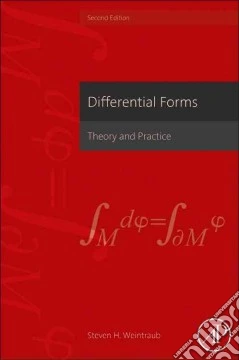ricerca
avanzata

# Differential Forms

## Un libro in lingua di Weintraub Steven H. edito da Academic Pr, 2014

• € 101,10
• Il prezzo è variabile in funzione del cambio della valuta d’origineDifferential forms are utilized as a mathematical technique to help students, researchers, and engineers analyze and interpret problems where abstract spaces and structures are concerned, and when questions of shape, size, and relative positions are involved. Differential Forms has gained high recognition in the mathematical and scientific community as a powerful computational tool in solving research problems and simplifying very abstract problems through mathematical analysis on a computer. Differential Forms, 2nd Edition, is a solid resource for students and professionals needing a solid general understanding of the mathematical theory and be able to apply that theory into practice. Useful applications are offered to investigate a wide range of problems such as engineers doing risk analysis, measuring computer output flow or testing complex systems. They can also be used to determine the physics in mechanical and/or structural design to ensure stability and structural integrity. The book offers many recent examples of computations and research applications across the fields of applied mathematics, engineering, and physics.

• The only reference that provides a solid theoretical basis of how to develop and apply differential forms to real research problems
• Includes computational methods for graphical results essential for math modeling
• Presents common industry techniques in detail for a deeper understanding of mathematical applications
• Introduces theoretical concepts in an accessible manner